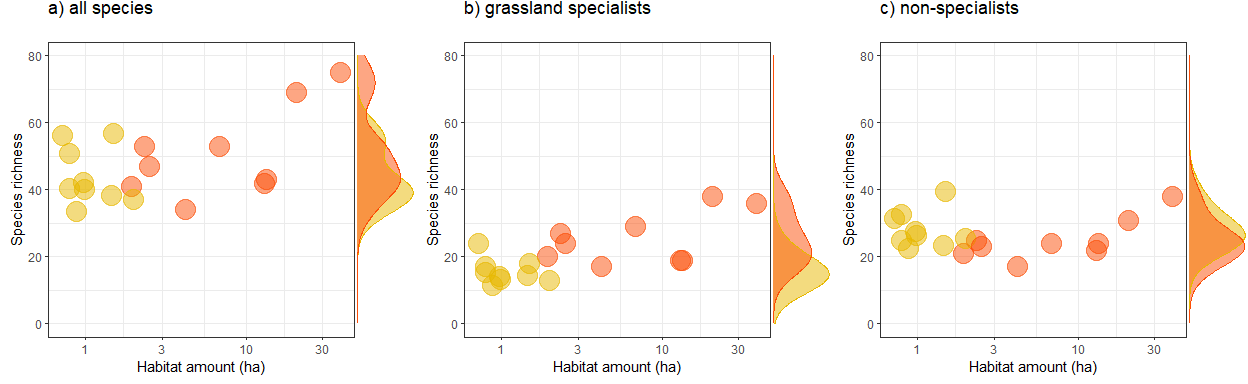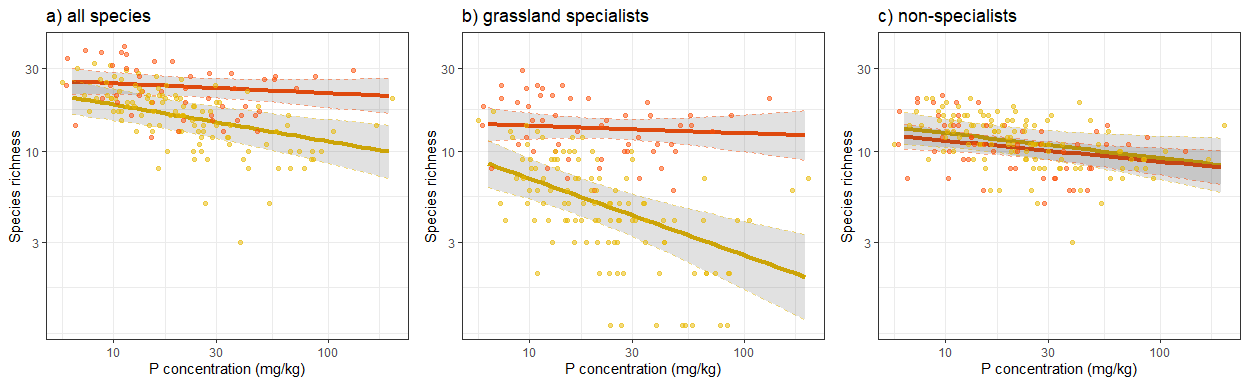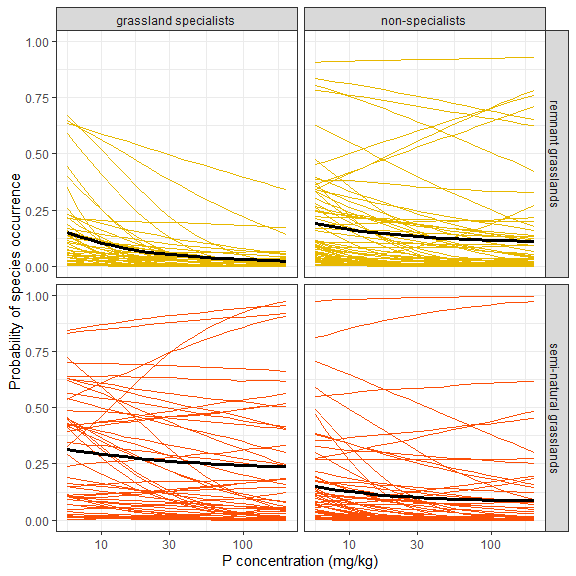## Soil phosphorous availability limits the contribution of small, individual grassland remnants to the conservation of landscape-scale biodiversity

Data analyses for Plue & Baeten (2021) Applied Vegetation Science

Here we provide code to reproduce the figures that appear in the main text of the study where we question under which conditions small remnant grasslands may exhibit the largest conservation value.

library(tidyverse)
library(nlme)
library(vegan)
library(ggExtra)
library(gridExtra)
library(Hmsc)

# function to trim lme outputs
trim <- function(model){
round(summary(model)$tTable, 3) } ### Data preparation Read the data from different .csv files and create some new variables that will be used in the analyses. Brief descriptions of the files and variables: • Soil data: soil.csv • P-Olsen (mg/kg), pH, N (%), and C (%) • Plant community data: vegdat.csv • abundance (class) of each species; note that for the analyses these were transformed to presence/absence data • Plot-level descriptors or meta-data: meta.csv • landscape name (Landscape) • grassland type (Type; MI = Midfield islet = remnant grassland, SNG = semi-natural grassland), • size of an MI as a class (Size; S = small, B = big) and as an actual size (Area; m²) • distance of an MI to the SNG as a class (Distance; 1 = closest to SNG, 3 = far from SNG) and as an actual distance (DistCont; km) • position of the plot in an MI (M = mid of the islet, E = towards the edge) • Plant traits: planttraits.csv • grassland specialists are species that decline after 5 or 10 years when grassland management stops (specialist_5 and specialist_10) • non-specialists are generalist or forest species soil <- read.csv("data/soil.csv", header = T) %>% rename(P = P_Olsen_mg.kg, pH = pH_H2O, N = N_percentage, C = C_percentage) vegdat <- read.csv("data/vegdat.csv", header = T, colClasses = "character") %>% gather(species, cover, Achillea.millefolium:Viola.sp.) %>% mutate(plot = as.integer(plot)) %>% mutate(pres = case_when( cover == "R" ~ 1, cover == "O" ~ 1, cover == "F" ~ 1, cover == "C" ~ 1, cover == "A" ~ 1, cover == "" ~ 0) ) %>% select(-cover) meta <- read.csv("data/plot_metadata.csv", header = T) TR <- read.csv("data/planttraits.csv", header = T) %>% mutate(species = gsub(" ", ".", species)) %>% mutate(specialist = specialist_5 + specialist_10) %>% mutate(specialist_name =ifelse(specialist == 1, "grassland specialists", "non-specialists")) Create a single dataset by joining the soil, vegetation, meta, and trait data. Then create an ID variable that identifies each patch, combining landscape name etc… One plot (ID = 161) showed extreme C percentage values, most probably due to wrong sampling in the field. This plot was removed before the analyses. dat <- vegdat %>% left_join(soil) %>% left_join(meta) %>% left_join(TR) %>% mutate(ID = case_when( Type == "MI" ~ paste(Landscape, Type, Size, Distance), Type == "SNG" ~ paste(Landscape, Type))) %>% mutate(Type = factor(Type, levels = c("SNG", "MI"))) %>% filter(plot != 161) head(dat) ### Landscape level richness patterns This is the analysis for research question 1 and Figure 2. First we calculate the habitat amount for remnant grasslands and semi-natural grasslands in every landscape. habitat_amount <- meta %>% group_by(Landscape, Type) %>% summarize(sum_area = sum(Area)) %>% # MI are counted twice this way (two plots per patch) # SNG are counted six times (same area around each plot) mutate(amount = ifelse(Type == "MI", sum_area/2, sum_area/6) / 10000) Calculate and test for differences in mean habitat amount between grassland types. habitat_amount %>% group_by(Type) %>% summarize(mn = round(mean(amount), 1))  trim(lme(log(amount) ~ Type, random=~ 1 | Landscape, data = habitat_amount))  Value Std.Error DF t-value p-value (Intercept) 0.055 0.265 8 0.206 0.842 TypeSNG 1.905 0.364 8 5.238 0.001 To account for the difference in the number of vegetation plots sampled in the semi-natural grasslands (n = 6 per landscape) and remnant grasslands (n = 12 per landscape), the species richness of the remnant grasslands needed to be rescaled. We calculated the average number of species across six plots, through sample-based rarefaction (n = 100 permutations). We run the same analyses on (1) all species, (2) the subset of specialists, and (3) the subset of non-specialists. To avoid code replication, we first create two helperfunctions: one for rarefaction and one for creating plots. # rarefaction function f_gamma <- function(vegdata){ # species accumulation curves spec_acc <- vegdata %>% group_map( ~ specaccum(comm = .x, method = "random", permutations = 100)) # extract mean richness across 6 plots gamma <- c() for(i in 1:18){ gamma[i] <- spec_acc[[i]]$richness
}

# only 5 SNG plots in Nyckleby
gamma <- spec_acc[]$richness # combine into data frame data.frame(gamma, group_keys(vegdata)) %>% left_join(habitat_amount) } # plot of landscape level richness f_plot_gamma <- function(gamma_out, title){ landscape_SR <- ggplot(gamma_out, aes(x = amount, y = gamma)) + geom_point(aes(color = Type), size = 7, alpha = 0.5) + scale_color_manual("", labels = c("remnant grasslands", "semi-natural grasslands"), values = c("#E7B800", "#FC4E07")) + scale_x_log10("Habitat amount (ha)") + scale_y_continuous("Species richness", limits = c(0,80)) + theme_bw() + theme(legend.position = "none") + ggtitle(title) ggMarginal(landscape_SR, groupColour = T, groupFill = T, margins = "y", bw = 5) } 1. Analysis on the full dataset, i.e. including all species Calculate richness vegdat_all <- dat %>% select(plot, Landscape, Type, species, pres) %>% spread(species, pres) %>% select(-plot) %>% group_by(Landscape, Type) gamma_out_all <- f_gamma(vegdat_all) Plot richness against habitat amount gamma_plot_all <- f_plot_gamma(gamma_out_all, "a) all species")  Test differences in richness between grassland types, either accounting or not accounting for habitat amount. trim(lme(gamma ~ scale(log(amount)) * Type, random=~ 1 | Landscape, data = gamma_out_all))  Value Std.Error DF t-value p-value (Intercept) 49.991 7.194 8 6.949 0.000 scale(log(amount)) 7.888 8.318 6 0.948 0.380 TypeSNG -7.514 7.221 6 -1.041 0.338 scale(log(amount)):TypeSNG 2.979 8.561 6 0.348 0.740 trim(lme(gamma ~ Type, random=~ 1 | Landscape, data = gamma_out_all))  Value Std.Error DF t-value p-value (Intercept) 43.966 3.759 8 11.696 0.000 TypeSNG 6.812 3.886 8 1.753 0.118 1. Analysis on the subset of specialist species Calculate richness vegdat_spec <- dat %>% filter(specialist_name == "grassland specialists") %>% select(plot, Landscape, Type, species, pres) %>% spread(species, pres) %>% select(-plot) %>% group_by(Landscape, Type) gamma_out_spec <- f_gamma(vegdat_spec) Plot richness against habitat amount gamma_plot_spec <- f_plot_gamma(gamma_out_spec, "b) grassland specialists") Test differences in richness between grassland types, either accounting or not accounting for habitat amount. trim(lme(gamma ~ scale(log(amount)) * Type, random=~ 1 | Landscape, data = gamma_out_spec))  Value Std.Error DF t-value p-value (Intercept) 15.471 4.899 8 3.158 0.013 scale(log(amount)) -0.240 5.935 6 -0.040 0.969 TypeSNG 6.106 5.164 6 1.182 0.282 scale(log(amount)):TypeSNG 5.303 6.149 6 0.862 0.422 trim(lme(gamma ~ Type, random=~ 1 | Landscape, data = gamma_out_spec))  Value Std.Error DF t-value p-value (Intercept) 15.654 2.015 8 7.769 0.000 TypeSNG 9.790 2.217 8 4.416 0.002 1. Analysis on the subset of non-specialist species Calculate richness vegdat_nonspec <- dat %>% filter(specialist_name == "non-specialists") %>% select(plot, Landscape, Type, species, pres) %>% spread(species, pres) %>% select(-plot) %>% group_by(Landscape, Type) gamma_out_nonspec <- f_gamma(vegdat_nonspec) Plot richness against habitat amount gamma_plot_nonspec <- f_plot_gamma(gamma_out_nonspec, "c) non-specialists") Test differences in richness between grassland types, either accounting or not accounting for habitat amount. trim(lme(gamma ~ scale(log(amount)) * Type, random=~ 1 | Landscape, data = gamma_out_nonspec))  Value Std.Error DF t-value p-value (Intercept) 31.366 4.835 8 6.487 0.000 scale(log(amount)) 4.089 5.913 6 0.692 0.515 TypeSNG -10.318 5.155 6 -2.001 0.092 scale(log(amount)):TypeSNG 1.085 6.147 6 0.177 0.866 trim(lme(gamma ~ Type, random=~ 1 | Landscape, data = gamma_out_nonspec))  Value Std.Error DF t-value p-value (Intercept) 28.242 1.922 8 14.696 0.000 TypeSNG -3.242 2.332 8 -1.390 0.202 Finally, we combine the plots for the three subsets of data into one multi-panel graph. grid.arrange(gamma_plot_all, gamma_plot_spec, gamma_plot_nonspec, nrow = 1)### Plot-level analyses #### Species richness patterns This is the analysis for research question 2 and Figure 3. Calculate the mean species richness in the two grassland types. dat %>% group_by(plot, Type) %>% summarize(SR = sum(pres)) %>% group_by(Type) %>% summarize(mn_SR = round(mean(SR), 1)) We run the same analyses on (1) all species, (2) the subset of specialists, and (3) the subset of non-specialists. To avoid code replication, we first create two helperfunctions: one for making predictions from the statistical model and one for creating plots. # predict along soil P gradient f_predict <- function(model){ # create new dataset with gradient in P newdat <- expand.grid(Type = c("MI","SNG"), pH_c = 0, CN_c = 0, P_c = seq(-1.2, 2.2, 0.1), fit = NA) # predictions and 95% CI's newdat$fit <- predict(model, newdata = newdat, level = 0)

des <- model.matrix(formula(model)[-2], newdat)
predvar <- diag( des %*% vcov(model) %*% t(des) )

newdat$lower = with(newdat, fit - 2*sqrt(predvar) ) newdat$upper = with(newdat, fit + 2*sqrt(predvar) )

# transform predictions to 'response' scale
# back-transform standardized log(P) to original soil P
# mean log(P) concentrations was 3.055
mn_log_P_resp <- 3.055

newdat %>%
mutate(fit_resp = exp(fit),
lower_resp = exp(lower),
upper_resp = exp(upper),
P_resp = exp(P_c) * exp(mn_log_P_resp)
)
}

# plot of plot-level richness
f_plot_SR <- function(SR_dat, SR_out, title){
ggplot(SR_out, aes(P_resp, fit_resp, col = Type)) +
geom_line(size = 1.5) +
geom_ribbon(aes(y = NULL, ymin = lower_resp, ymax = upper_resp), linetype = 0, alpha = 0.15) +
geom_line(aes(P_resp, lower_resp), linetype = 2, alpha = 0.5) +
geom_line(aes(P_resp, upper_resp), linetype = 2, alpha = 0.5) +
geom_point(data = SR_dat, aes(P, SR+1), alpha = 0.5) +
ggtitle(title) +
theme_bw() +
theme(legend.position = "none") +
scale_x_log10("P concentration (mg/kg)") +
scale_y_continuous("Species richness", trans = "log", breaks = c(3, 10, 30), limits = c(1,40)) +
scale_color_manual(values = c("#E7B800", "#FC4E07"))
}
1. Analysis on the full dataset, i.e. including all species

Fit model

dat_SR_all <- dat %>%
mutate(CN_c = C/N -  mean(C/N),
P_c = log(P) - mean(log(P)),
pH_c = pH - mean(pH, na.rm = T)) %>%
group_by(plot, ID, Landscape, P, P_c, pH_c, CN_c, Type) %>%
summarize(SR = sum(pres))

m_SR_all <- lme(log(SR+1) ~ 1 + Type * (P_c + pH_c + CN_c),
random=~ 1 | Landscape/ID, data = dat_SR_all)
trim(m_SR_all)
             Value Std.Error DF t-value p-value
(Intercept)  3.156     0.100 92  31.648   0.000
TypeMI      -0.395     0.093 53  -4.239   0.000
P_c         -0.057     0.060 92  -0.941   0.349
pH_c         0.109     0.196 92   0.556   0.580
CN_c         0.023     0.031 92   0.737   0.463
TypeMI:P_c  -0.155     0.074 92  -2.100   0.038
TypeMI:pH_c -0.093     0.215 92  -0.432   0.667
TypeMI:CN_c  0.016     0.037 92   0.439   0.662

Predictions and plot

pred_all <- f_predict(m_SR_all)
SR_plot_all <- f_plot_SR(dat_SR_all, pred_all, "a) all species")
1. Analysis on the subset of specialist species

Fit model.

dat_SR_spec <- dat %>%
filter(specialist_name == "grassland specialists") %>%
mutate(CN_c = C/N -  mean(C/N),
P_c = log(P) - mean(log(P)),
pH_c = pH - mean(pH, na.rm = T)) %>%
group_by(plot, ID, Landscape, P, P_c, pH_c, CN_c, Type) %>%
summarize(SR = sum(pres))

m_SR_spec <- lme(log(SR+1) ~ 1 + Type * (P_c + pH_c + CN_c),
random=~ 1 | Landscape/ID, data = dat_SR_spec)
trim(m_SR_spec)
             Value Std.Error DF t-value p-value
(Intercept)  2.606     0.129 92  20.140   0.000
TypeMI      -0.997     0.136 53  -7.325   0.000
P_c         -0.043     0.102 92  -0.418   0.677
pH_c         0.344     0.335 92   1.029   0.306
CN_c         0.073     0.054 92   1.343   0.183
TypeMI:P_c  -0.397     0.122 92  -3.258   0.002
TypeMI:pH_c -0.361     0.362 92  -0.998   0.321
TypeMI:CN_c  0.068     0.063 92   1.074   0.286

Predictions and plot.

pred_spec <- f_predict(m_SR_spec)
SR_plot_spec <- f_plot_SR(dat_SR_spec, pred_spec, "b) grassland specialists")
1. Analysis on the subset of non-specialist species

Fit model.

dat_SR_nonspec <- dat %>%
filter(specialist_name == "non-specialists") %>%
mutate(CN_c = C/N -  mean(C/N),
P_c = log(P) - mean(log(P)),
pH_c = pH - mean(pH, na.rm = T)) %>%
group_by(plot, ID, Landscape, P, P_c, pH_c, CN_c, Type) %>%
summarize(SR = sum(pres))

m_SR_nonspec <- lme(log(SR+1) ~ 1 + Type * (P_c + pH_c + CN_c),
random=~ 1 | Landscape/ID, data = dat_SR_nonspec)
trim(m_SR_nonspec)
             Value Std.Error DF t-value p-value
(Intercept)  2.353     0.091 92  25.968   0.000
TypeMI       0.083     0.083 53   0.995   0.324
P_c         -0.120     0.063 92  -1.914   0.059
pH_c        -0.197     0.205 92  -0.958   0.341
CN_c        -0.029     0.033 92  -0.885   0.378
TypeMI:P_c  -0.023     0.075 92  -0.308   0.759
TypeMI:pH_c  0.215     0.222 92   0.972   0.334
TypeMI:CN_c  0.039     0.039 92   0.996   0.322

Predictions and plot.

pred_nonspec <- f_predict(m_SR_nonspec)
SR_plot_nonspec <- f_plot_SR(dat_SR_nonspec, pred_nonspec, "c) non-specialists")

Finally, we combine the plots for the three subsets of data into one multi-panel graph.

grid.arrange(SR_plot_all, SR_plot_spec, SR_plot_nonspec, nrow = 1)#### Community composition patterns

This is the analysis for research question 3 and Figure 4.

First we prepare the data input for the species community model.

# select multivariate species data
Y_dat <- dat %>%
select(plot, species, pres) %>%
select(-plot)

Y_dat_freq <- Y_dat[, colSums(Y_dat) > 2]

Y <- data.matrix(Y_dat_freq)

# environmental predictors
env <- dat %>%
select(plot, Type, P, pH, N, C) %>%
mutate(CN_c = C/N -  mean(C/N),
P_c = log(P) - mean(log(P)),
pH_c = pH - mean(pH, na.rm = T)) %>%
group_by(plot, Type) %>%
summarize(P = mean(P_c),
CN = mean(CN_c),
pH = mean(pH_c))

XData <- env %>%
group_by() %>%
select(-plot)

XData <- as.data.frame(XData)

# plant traits
TrData <- dat %>%
filter(species %in% colnames(Y)) %>%
select(species, specialist_name) %>%
distinct() %>%
column_to_rownames("species")

# spatio-temporal context
studyDesign <- data.frame(patch = factor(dat$ID[1:161]), landscape = factor(dat$Landscape[1:161]))
rL <- HmscRandomLevel(units = studyDesign$pach) rL2 <- HmscRandomLevel(units = studyDesign$landscape)

rm(Y_dat, Y_dat_freq, env)

Code to set-up and run the HMSC model. Note that it takes few hours of sampling. So you only want to do this once and save the output. This way, you could reload the model output whenever needed. This was also done here.

m <- Hmsc(Y = Y,
XData = XData, XFormula = ~ Type * (P + pH + CN),
TrData = TrData, TrFormula = ~ specialist_name,
studyDesign = studyDesign,
ranLevels = list(patch = rL, landscape = rL2), distr = "probit")

# only run next lines when you want to resample the model
# m <- sampleMcmc(m, thin = 10, samples = 1000, transient = 500, nChains = 2, verbose = 500)
# save(m, "output/hmsc_out.RData")

load("output/hmsc_out.RData")

To make predictions from this model, we first construct a gradient in soil P, while keeping the other predictors at a constant value.

Gradient_MI = constructGradient(m,focalVariable = "P",
non.focalVariables = list("Type" = list(3, "MI"),
"pH" = list(3, 0.38),
"CN" = list(3, 0)))

non.focalVariables = list("Type" = list(3, "SNG"),
"pH" = list(3, 0.38),
"CN" = list(3, 0)))

Make predictions. Then, wrangle the output for creating a plot.

predY_MI = predict(m, XData=Gradient_MI$XDataNew, studyDesign=Gradient_MI$studyDesignNew,
ranLevels=Gradient_MI$rLNew, expected=TRUE) predY_SNG = predict(m, XData=Gradient_SNG$XDataNew, studyDesign=Gradient_SNG$studyDesignNew, ranLevels=Gradient_SNG$rLNew, expected=TRUE)

predY_MI_bind <- abind::abind(predY_MI, along = 3)
qpredY_MI <- apply(predY_MI_bind, c(1, 2), quantile, probs = c(.5), na.rm = TRUE) %>%
as.data.frame() %>%
mutate(P = Gradient_MI$XDataNew$P,
type = "MI") %>%
gather(species, pred, 1:115) %>%
left_join(TR) %>%
select(P, type, species, pred, specialist_name)

predY_SNG_bind <- abind::abind(predY_SNG, along = 3)
qpredY_SNG <- apply(predY_SNG_bind, c(1, 2), quantile, probs = c(.5), na.rm = TRUE) %>%
as.data.frame() %>%
mutate(P = Gradient_SNG$XDataNew$P,
type = "SNG") %>%
gather(species, pred, 1:115) %>%
left_join(TR) %>%
select(P, type, species, pred, specialist_name)

qpredY <- rbind(qpredY_MI, qpredY_SNG)

qpredY_summary <- qpredY %>%
group_by(P, specialist_name, type) %>%
summarize(mn_pred = mean(pred))

Back-transform standardised log(P) to original soil P and create the plot.

# Back-transform standardized P values
mn_log_P_resp <- mean(log(dat$P)) qpredY$P_resp <- exp(qpredY$P) * exp(mn_log_P_resp) qpredY_summary$P_resp <- exp(qpredY_summary$P) * exp(mn_log_P_resp) type_labs <- c("remnant grasslands", "semi-natural grasslands") names(type_labs) <- c("MI", "SNG") ggplot(qpredY, aes(P_resp, pred, col = type)) + geom_line(aes(group = species)) + geom_line(data = qpredY_summary, aes(P_resp, mn_pred), size = 1.1, colour = "black") + facet_grid(type ~ specialist_name, labeller = labeller(type = type_labs)) + theme_bw() + theme(legend.position = "none") + scale_x_log10("P concentration (mg/kg)") + scale_y_continuous("Probability of species occurrence") + scale_color_manual("", labels = c("remnant grasslands", "semi-natural grasslands"), values = c("#E7B800", "#FC4E07"))#### Variance partitioning Analysis for research question 4 and Figure 5. Using the output from the species community model, we can calculate the relative importance of the different predictors. We do this for the two grassland types separately. 1. semi-natural grasslands VP_SNG = computeVariancePartitioning(m, group = c(1,0,2,2,2), groupnames=c("type","abiotic")) variances_SNG <- data.frame(factor = rownames(VP_SNG$vals), VP_SNG$vals, row.names = NULL) %>% tidyr::gather(species, value, 2:116) %>% left_join(TR) %>% mutate(factor = factor(factor, levels = c("type", "abiotic", "Random: landscape","Random: patch"), labels = c("type", "abtioic", "landscape","patch"))) variances_summary_SNG <- variances_SNG %>% group_by(specialist, factor) %>% summarize(n = n_distinct(species), mn_value = mean(value, na.rm = T), se_value = sd(value, na.rm = T)/sqrt(n)) VARS_graph_a <- ggplot(variances_summary_SNG, aes(factor(specialist), mn_value, col = factor)) + geom_point(position = position_dodge(width = 0.4)) + geom_errorbar(aes(ymin = mn_value - 2 * se_value, ymax = mn_value + 2 * se_value), position = position_dodge(width = 0.4), width = 0.5) + theme_bw() + scale_x_discrete("", labels = c("non-specialists","grassland specialists")) + scale_y_continuous("Variance proportion", limits = c(0, 0.8)) + scale_colour_discrete("") + ggtitle("a) semi-natural grasslands") + theme(legend.position = "none") 1. remnant grasslands VP_MI = computeVariancePartitioning(m, group = c(1,1,2,2,2,2,2,2), groupnames=c("type","abiotic")) variances_MI <- data.frame(factor = rownames(VP_MI$vals), VP_MI\$vals, row.names = NULL) %>%
tidyr::gather(species, value, 2:116) %>%
left_join(TR) %>%
mutate(factor = factor(factor, levels = c("type", "abiotic", "Random: landscape","Random: patch"),
labels = c("type", "abtioic", "landscape","patch")))

variances_summary_MI <- variances_MI %>%
group_by(specialist, factor) %>%
summarize(n = n_distinct(species),
mn_value = mean(value, na.rm = T),
se_value = sd(value, na.rm = T)/sqrt(n))

VARS_graph_b <- ggplot(variances_summary_MI, aes(factor(specialist), mn_value, col = factor)) +
geom_point(position = position_dodge(width = 0.4)) +
geom_errorbar(aes(ymin = mn_value - 2 * se_value, ymax = mn_value + 2 * se_value), position = position_dodge(width = 0.4), width = 0.5) +
theme_bw() +
scale_x_discrete("", labels = c("non-specialists","grassland specialists")) +
scale_y_continuous("", limits = c(0, 0.8)) +
scale_colour_discrete("") +
ggtitle("b) midfield islets") +
theme(legend.margin = margin(.9, .9, .9, .9), legend.position = c(0.8, 0.82))

Arrange the two graphs into a multipanel figure.

grid.arrange(VARS_graph_a, VARS_graph_b, nrow = 1)